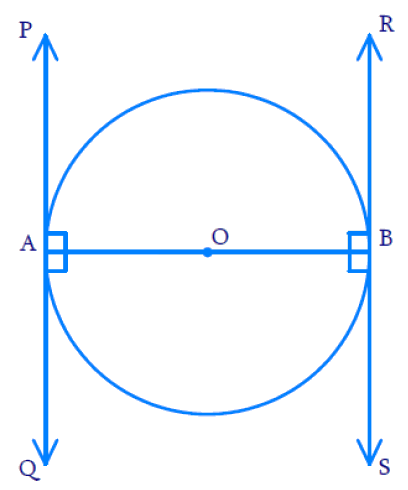# Ex.10.2 Q4 Circles Solution - NCERT Maths Class 10

Go back to  'Ex.10.2'

## Question

Prove that the tangents drawn at the ends of a diameter of a circle are parallel.

Video Solution
Circles
Ex 10.2 | Question 4

## Text SolutionWhat is known?

• Let $$AB$$ be a diameter of the circle.
• Two tangents $$PQ$$ and $$RS$$ are drawn at points $$A$$ and $$B$$ respectively.

To Prove:

Tangents drawn at the ends of a diameter of a circle are parallel.

Reasoning :

• A tangent to a circle is a line that intersects the circle at only one point.
• Theorem 10.1 : The tangent at any point of a circle is perpendicular to the radius through the point of contact.

Steps:

We know that, according to Theorem 10.1, Radius is perpendicular to the tangent at the point of contact

Thus, $${OA}\, \bot \, {PQ}$$ and $${OB}\, \bot\, {Z}$$

Since the Tangents are Perpendicular to the Radius,

\begin{aligned} \angle {P A O} & = 90 ^ { \circ } \\ \angle {R B O} & = 90 ^ { \circ } \\ \angle {O A Q} & = 90 ^ { \circ } \\ \angle {O B S} & = 90 ^ { \circ } \end{aligned}

Here $$\angle {OAQ}$$ & $$\angle {OBR}$$ and $$\angle {PAO}$$ & $$\angle {OBS}$$ are two pairs of alternate interior angles and they are equal.

If the Alternate interior angles are equal then lines $$PQ$$ and $$RS$$ should be parallel.

We know that $$PQ$$ & $$RS$$ are the tangents drawn to the circle at the ends of the diameter $$A B.$$

Hence, it is proved that Tangents drawn at the ends of a diameter of a circle are parallel.

Learn from the best math teachers and top your exams

• Live one on one classroom and doubt clearing
• Practice worksheets in and after class for conceptual clarity
• Personalized curriculum to keep up with school